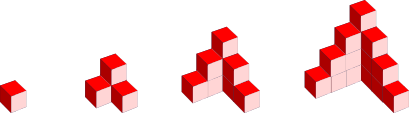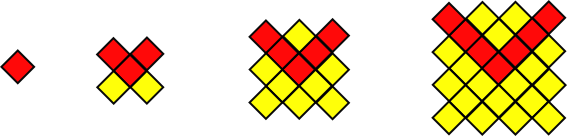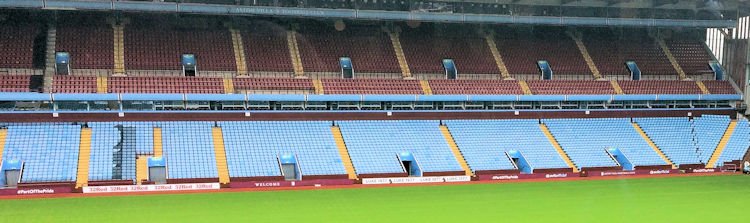# Exam-Style Questions.

## Problems adapted from questions set for previous Mathematics exams.

### 1.

GCSE Higher

The first five terms of an arithmetic sequence are:

$$-20, -17, -14, -11, -8$$

Find an expression for the $$n^{th}$$ term of the sequence.

### 2.

GCSE Higher

Here is a picture of four models. Some of the cubes are hidden behind other cubes.Model one consists of one cube. Model two consists of four cubes and so on.

(a) How many cubes are in the third model?

(b) How many cubes are in the fourth model?

(c) If a fifth model were built, how many cubes would it take?

(d) Find an expression for the number of cubes used in the nth model.

(e) Sketch a side view, front view and plan view of the fourth model.

### 3.

GCSE Higher

This expression can be used to generate a sequence of numbers.

$$n^2+n + 5$$

(a) Work out the first three terms of this sequence.

(b) What is the smallest value of $$n$$ that produces a term of the sequence that is not a prime number?

(c) Is it true that odd square numbers have exactly three factors? Explain and justify your answer.

(d) Seymour is thinking of a number.

• It is a common factor of 144 and 192.
• It is a common multiple of 6 and 8.
• It is less than 100.

Find the two possible numbers that Seymour could be thinking of.

### 4.

GCSE Higher

Work out an expression for the nth term of the quadratic sequence

$$0,9,22,39,60, ...$$

Give your answer in the form $$an^2 + bn + c$$ where a, b and c are constants.

### 5.

GCSE Higher

Here are the first six terms of a quadratic sequence.

$$-1, 8, 21, 38, 59, 84$$

Find an expression, in terms of $$n$$, for the $$n$$th term of this sequence.

### 6.

GCSE Higher

Find an expression, in terms of $$n$$, for the $$n$$th term of the sequence that has the following first five terms:

$$6 \qquad 13 \qquad 23 \qquad 36 \qquad 52$$

### 7.

IGCSE ExtendedThe diagrams above show a growing fractal of triangles. The sides of the largest equilateral triangle in each diagram are of length 1 metre.

In the second diagram there are four triangles each with sides of length $$\frac{1}{2}$$ metre.

In the third diagram there are 16 triangles each with sides of length $$\frac{1}{4}$$ metre.

(a) Complete this table for more diagrams.

 Diagram 1 Diagram 2 Diagram 3 Diagram 4 Diagram 5 Diagram 6 Diagram $$n$$ Length of Side 1 $$\frac{1}{2}$$ $$\frac{1}{4}$$ Power of 2 20 2-1 2-2

(b) Complete this table for the number of the smallest triangles in diagrams 4, 5 and 6.

 Diagram 1 Diagram 2 Diagram 3 Diagram 4 Diagram 5 Diagram 6 Diagram $$n$$ Number of smallest triangles 1 4 16 Power of 2 20 22 24

(c) Calculate the number of the smallest triangles in the diagram where the smallest triangles have sides of length $$\frac{1}{256}$$ metre.

### 8.

GCSE Higher

The diagrams below show a sequence of patterns made from red and yellow tiles.(a) Find an expression, in terms of $$n$$, for the number of red tiles in pattern $$n$$.

The total number of red and yellow tiles in each pattern is always the sum of the squares of two consecutive whole numbers.

(b) Find an expression, in terms of $$n$$, for the total number tiles in Pattern $$n$$ giving your answer in its simplest form.

(c) Is there a pattern for which the total number of tiles is 303? Give a reason for your answer.

(d) Explain why the total number of tiles in any pattern of this sequence is always an odd number.

### 9.

IB Applications and Interpretation

A celebrity football match is planned to take place in a large stadium.The most expensive tickets are in the first row. The ticket price for each row forms an arithmetic sequence. Prices for the first four rows are shown in the following table.

 Row number: Price per seat 1 2 3 4 £50 £48.50 £47 £45.50

(a) Write down the value of the common difference, $$d$$.

(b) Calculate the price of a ticket in the 19th row.

(c) Find the total cost of buying 5 tickets in each of the first 10 rows.

If you would like space on the right of the question to write out the solution try this Thinning Feature. It will collapse the text into the left half of your screen but large diagrams will remain unchanged.

The exam-style questions appearing on this site are based on those set in previous examinations (or sample assessment papers for future examinations) by the major examination boards. The wording, diagrams and figures used in these questions have been changed from the originals so that students can have fresh, relevant problem solving practice even if they have previously worked through the related exam paper.

The solutions to the questions on this website are only available to those who have a Transum Subscription.

Exam-Style Questions Main Page

Search for exam-style questions containing a particular word or phrase:

To search the entire Transum website use the search box in the grey area below.